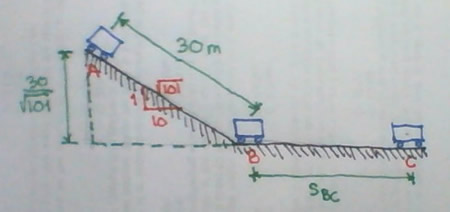# another problem :(

2 posts / 0 new
Kenneth Bryan M...another problem :(

a car if nass 1600kg coast down a 10 percent grade from A to B, a distance of 30m and then coast along a level road until it stops at C. assume the road resistance to remain constant of 200N for the entire distance A to C, if the initial velocity of the car at A is 25kph, compute:

a.) its velocity at B
b.) the distance s it coast from B to C.

Jhun VertMotion from A to B

EA - Friction Work = EB
$\left[ \frac{1}{2}(1600)\left( \dfrac{25}{3.6} \right)^2 + 1600(9.81) \left( \dfrac{30}{\sqrt{101}} \right) \right] - 200(30) = E_B$

$E_B = 79,434.56 ~ \text{N}\cdot\text{m}$

$\frac{1}{2}(1600)\left( \dfrac{v_B}{3.6} \right)^2 = 79,434.56$

$v_B = 35.87 ~ \text{kph}$Motion from B to C

EB - Friction Work = EC
$79,434.56 - 200s_{BC} = 0$

$s_{BC} = 397.17 ~ \text{m}$

Subscribe to MATHalino on